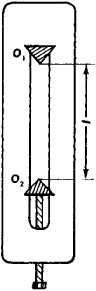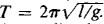# Pendulum, Reversible

The following article is from The Great Soviet Encyclopedia (1979). It might be outdated or ideologically biased.

## Pendulum, Reversible

an instrument for experimental determination of the acceleration of gravity g. A reversible pendulum consists of a body, such as a solid plate, and two trihedral knives (Figure 1). One of the knives is stationary; the other knife can be moved along a slot in the plate. The sharp edges of the knives O1 and O2 can be alternately placed on a rigid support. The supported knife edge becomes the axis of oscillation of the reversible pendulum. The movable knife is moved up or down until the periods of oscillation, which are measured with a stopwatch, become equal for both axes.Figure 1. Reversible pendulum

The distance O1, O2 = l between the two axes can be measured with the aid of a vernier scale marked on the plate. In this case, as is known from the properties of a compound pendulum, O2 becomes the center of oscillation for O1 and vice versa, and the period of small-amplitude oscillations equalsSince the values of T and l can be determined experimentally, the value of g can be computed from the formula. Use of a reversible pendulum makes it possible to determine the value of g with a much higher degree of precision than that obtained with a simple pendulum.

The Great Soviet Encyclopedia, 3rd Edition (1970-1979). © 2010 The Gale Group, Inc. All rights reserved.
Site: Follow: Share:
Open / Close